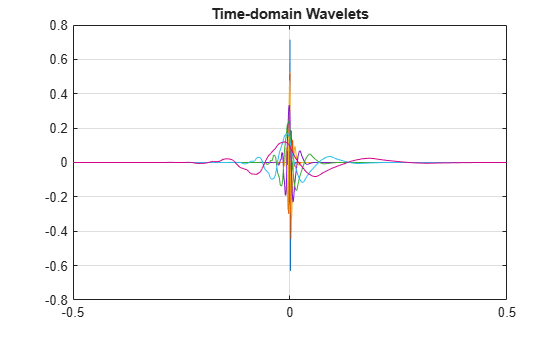# wavelets

DWT filter bank time-domain wavelets

## Syntax

``psi = wavelets(fb)``
``[psi,t] = wavelets(fb)``

## Description

````psi = wavelets(fb)` returns the time-domain and centered wavelets corresponding to the wavelet passband filters in the discrete wavelet transform (DWT) filter bank `fb`.```

example

````[psi,t] = wavelets(fb)` returns the sampling instants `t`.```

## Examples

collapse all

Create a seven-level DWT filter bank with a signal length of 1000 samples, using the Daubechies `db2` wavelet and a sampling frequency of 1 kHz.

```wv = "db4"; len = 1000; lev = 7; Fs = 1e3; fb = dwtfilterbank('Wavelet',wv,'SignalLength',len,'Level',lev,'SamplingFrequency',Fs);```

Plot the time-domain and centered wavelets corresponding to the wavelet bandpass filters.

```[psi,t] = wavelets(fb); plot(t,psi') grid on title('Time-domain Wavelets')```Plot the finest scale time-domain wavelet and the one-sided magnitude frequency response of the corresponding wavelet bandpass filter.

```sc = 1; [psidft,f] = freqz(fb); subplot(2,1,1) plot(t,psi(sc,:)) grid on xlabel('Time (sec)') ylabel('Magnitude') title(['Level ',num2str(sc),' Time-Domain Wavelet']) subplot(2,1,2) plot(f(len/2:end),abs(psidft(sc,len/2:end))) grid on xlabel('Hz') ylabel('Magnitude') title('Magnitude Frequency Response')```## Input Arguments

collapse all

Discrete wavelet transform (DWT) filter bank, specified as a `dwtfilterbank` object.

## Output Arguments

collapse all

Time-centered wavelets corresponding to the wavelet passband filters, returned as an L-by-N matrix, where L is the filter bank `Level` and N is the `SignalLength`. The wavelets are ordered in `psi` from the finest scale resolution to the coarsest scale resolution.

Sampling instants, returned as a real-valued vector `t` of length N, where N is the filter bank `SignalLength`. Sampling instants lie in the interval $\left[-\text{\hspace{0.17em}}½\text{\hspace{0.17em}}N\text{ }DT,\text{\hspace{0.17em}}\text{\hspace{0.17em}}½\text{\hspace{0.17em}}N\text{ }DT\right)$, where DT is the filter bank sampling period (reciprocal of the filter bank sampling frequency).